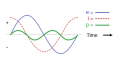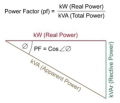# Capacitor as current limiter. Where does the energy go?

#### Rufinus

Joined Apr 29, 2020
225
Hello.

I know you can use a capacitor to limit AC current due its capacitive impedance. For example if I connect to the wall socket at 110V 60hz a 200 microfarad ideal capacitor the equivalent resistance will be around 13 ohms, so the current will be around 8 amps. 110x8 = 880 wats. But an ideal capacitor don´t disipate energy, so, I´m paying for that energy? And where does this energy goes? If it was a resistor, it would generate heat, but the capacitor??

Thank you

Best regards

#### crutschow

Joined Mar 14, 2008
32,926
Power is the voltage times current at any instant in time.
In a resistor the current and voltage are in phase, so real power is dissipated.
In a capacitor the voltage and current are 90° out of phase so no real power is dissipated (it's called reactive power).
The only real power dissipated is from the reactive current through the resistance of the power line wires.

The power meter to your residence only measures real power, not reactive power, so you don't pay for the reactive power.

•xox and DickCappels

#### Rufinus

Joined Apr 29, 2020
225

#### nsaspook

Joined Aug 27, 2009
11,794
The energy of the circuit is not in the current, it's in the fields surrounding the wires and in the electric field between the plates of the capacitor. As was said before there is a phase shift between the fields in your circuit so the reactive filed energy just flows in and flows out (instantaneous power may be positive or negative) instead of moving moving from source to load. The electrical energy generation source (generator) must still supply this energy, so large industrial users (not residential users) with lots of reactive devices like motors get charged for the reactive power draw as an incentive to them to correct the Power Factor of the plant as the current related resistance voltage drops are real power losses and real losses to the utility.

•Rufinus

#### crutschow

Joined Mar 14, 2008
32,926
The electrical energy generation source (generator) must still supply this energy
To be clear, the net real energy from the generator to provide this reactive power is zero.

Last edited:
•Rufinus

#### ApacheKid

Joined Jan 12, 2015
1,269
Ahh right, thank you!
The power is both positive and negative, that is energy moves from the capacitor to the load and back again (so the "net" power is zero)Don't focus on "impedance" here either, focus on reactance first, that is the property you deal with for an isolated capacitor (or inductor) impedance is appropriate only when both capacitance AND inductance is present.

•Rufinus

#### nsaspook

Joined Aug 27, 2009
11,794
To be clear, the net real energy from the generator to provide this reactive power is zero.
That reactive energy travels down power lines where some portion of that circulating reactive power is converted into extra (a higher current is required to deliver the actual power to the load) real resistive (extra EM field energy converted to conduction charge carrier KE as mainly thermal energy) power losses, so for big industrial users it matters to have the correction as close to the reactive load as possible. Homes don't get charged but there are rules on consumer products to have PF correction to minimize home reactive supply power utility losses.

The conductor(s) (in the generator and distribution) sees it just as current, it doesn't split the real and reactive parts. Real, reactive, complex power are parts of a simplified math model for easily (and usually totally satisfying) calculation of EM field energy in a circuit. The Poynting vector represents the power flow of an electromagnetic field. Real and imaginary parts of the electromagnetic fields are simplifications.

https://electrocorder.com/pages/knowledge-power-factor-correctionIn an electric power system, a load with a low power factor (tending towards zero) draws more current than a load with a high power factor (tending towards one) for the same amount of useful power transferred. The higher currents increase the energy lost in the distribution system and require larger wires and other equipment. Because of the costs of larger equipment and wasted energy, electrical utilities will usually charge a higher cost to industrial or commercial customers where there is a low power factor.

Last edited:
•Rufinus

#### Rufinus

Joined Apr 29, 2020
225

So if for example, I have a resistive wire to make a heat element, of 18 ohms and I want it to deliver 1.150 wats , I´ll need a current of P = I^2 x R I = 8 Amps. But since I´ll connect the resistance to the socket, at 230V 50hz, I would need a capacitor in series to limit the current. To get a current of 8 Amps, the total resistance in the circuit (Heatin resistance and capacitor) has to be 230V/8A = 29ohm, so since the heating resistance is 18 ohm, I´ll need a capacitaro that behaves as a 11 ohms resistor. My calculations give me 288 uF capacitor. Is that correct?

Thank you

#### nsaspook

Joined Aug 27, 2009
11,794
•Rufinus

#### Rufinus

Joined Apr 29, 2020
225

#### WBahn

Joined Mar 31, 2012
29,173
The power is both positive and negative, that is energy moves from the capacitor to the load and back again (so the "net" power is zero)

View attachment 294183

Don't focus on "impedance" here either, focus on reactance first, that is the property you deal with for an isolated capacitor (or inductor) impedance is appropriate only when both capacitance AND inductance is present.
Impedance is a perfectly correct term. Impedance has a resistive component and a reactive component, either of which can happen to be zero.

If you have both capacitance and inductance, you still don't need impedance and can just use reactance since capacitive reactance is negative while inductive reactance is positive.

•Rufinus

#### WBahn

Joined Mar 31, 2012
29,173

So if for example, I have a resistive wire to make a heat element, of 18 ohms and I want it to deliver 1.150 wats , I´ll need a current of P = I^2 x R I = 8 Amps. But since I´ll connect the resistance to the socket, at 230V 50hz, I would need a capacitor in series to limit the current. To get a current of 8 Amps, the total resistance in the circuit (Heatin resistance and capacitor) has to be 230V/8A = 29ohm, so since the heating resistance is 18 ohm, I´ll need a capacitaro that behaves as a 11 ohms resistor. My calculations give me 288 uF capacitor. Is that correct?

Thank you
It's not quite that simple, but you have the basic idea.

Resistances and reactances in series don't add up like two resistances (or two reactances) in series would. This is due to that phase shift in the voltage-current relationships.

You want the total impedance of the circuit to result in 8 A_rms through 230 V_rms, so need an impedance whose magnitude is 29 Ω. But resistance and reactance add like the sides of a right triangle to give an impedance whose magnitude is the length of the hypotenuse. So to achieve 29 Ω of impedance, you would need about 23 Ω of reactance. So that would be about 138 µF of capacitance at 50 Hz.

But it gets a bit more complicated, because heating elements tend to have resistances that change considerably depending on what their actual temperature is. So that 18 Ω is the resistance of your heating element at one particular temperature, which is probably not the temperature it will be at when it happens to be dissipating 1150 W.

•Rufinus, xox and nsaspook

#### Rufinus

Joined Apr 29, 2020
225

#### MrAl

Joined Jun 17, 2014
10,612
Hello.

I know you can use a capacitor to limit AC current due its capacitive impedance. For example if I connect to the wall socket at 110V 60hz a 200 microfarad ideal capacitor the equivalent resistance will be around 13 ohms, so the current will be around 8 amps. 110x8 = 880 wats. But an ideal capacitor don´t disipate energy, so, I´m paying for that energy? And where does this energy goes? If it was a resistor, it would generate heat, but the capacitor??

Thank you

Best regards
Hi,

As you probably know now, the capacitor charges and discharges. When it charges it takes energy from the line, and when it discharges it puts energy back into the line. As time goes on, energy goes into the cap then back into the line. If everything was perfect, all the energy that went into the cap would go back into the line and so the cost to operate would be zero.

That's not really the case though, because everything is not perfect. There is resistance in the wires and the cap is not perfect either, so you end up paying a little for the energy lost in the process of charging and discharging the capacitor which requires current to flow though wires which are resistive and resistive elements dissipate energy as heat and that is, in most cases, not recoverable.
You can figure out how much it costs by figuring out what the resistance of the wire is and what the current is. The power is the current squared times the resistance. You have to pay for that, but for normal sized capacitors it should not be very much.

•Rufinus and nsaspook